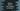# Python program to check if a set contains an element or not## Python program to check if a set contains an element or not:

In this post, we will learn how to check if a Python set contains a specific element or not. Set is a built-in data type in Python. It is used to store unindexed and unordered unique elements. We can’t add duplicate elements to a set.

### Example of set:

sets are written using curly braces. For example,

``given_set = {'a', 'b', 'c'}``

We can use the add method to add an element to a set. For example,

``````given_set = {'a', 'b', 'c'}

print(given_set)``````

We are adding c. Since it already exists in the set, it will not add again.

It will print:

``{'a', 'b', 'c'}``

### Check if a set contains an element or not in Python:

We can simply use the in keyword to check if a set contains an element or not in Python. The in keyword is used in many places in Python. For example, we can use it to check if an element is in other sequences like a list, or we can use in with a for loop etc.

It returns a boolean value if we use it with a set. If the element is in the set, it returns True, else it returns False.

Let me show you with an example:

``````given_set = {'a', 'b', 'c'}

print('a' in given_set)
print('d' in given_set)``````

### Using in with if-else:

Since in returns a boolean value, we can use it with a if-else block to check if an element is in a set or not:

``````vowels = {'a', 'e', 'i', 'o', 'u'}

v = input('Enter a character: ')

if v in vowels:
print('It is a vowel')
else:
print('It is not a vowel')``````

In this program, vowels is a set of vowels. We are asking the user to enter a character and storing that value in v.

The if block is checking if the entered character is in the set or not. Since the set contains only vowels, if the character is in the set, it will return True. Else, it will return False.

Based on the result, it is printing a message.

It will print output as like below:

``````Enter a character: e
It is a vowel

Enter a character: x
It is not a vowel``````

### Using not in operator to check if an element is in a set or not:

We can also use not in operator to check if an element is in a set or not. This is exactly opposite to the in operator, i.e. if the element is in the set, it returns False, else it returns True.

For example:

``````vowels = {'a', 'e', 'i', 'o', 'u'}

print('a' not in vowels)
print('x' not in vowels)``````

It will print:

``````False
True``````

Let’s write the above vowel checker program using not in:

``````vowels = {'a', 'e', 'i', 'o', 'u'}

v = input('Enter a character: ')

if v not in vowels:
print('It is not a vowel')
else:
print('It is a vowel')``````

As you can see, we have to reverse the print statements to make the program work with not in. If you run this program, it will print similar output.